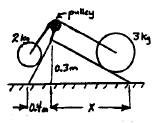# Balancing two rollers on a hill

## Homework Statement

Two rollers, of masses 2kg and 3kg, are connected over a pulley by a massless string. They rest in equilibrium on two inclined slopes, as shown in the diagram to the left. Friction is negligible. What is the distance X?## Homework Equations

Fg = mg
Component of Fg parallel to the slope = mgsin(theta)

## The Attempt at a Solution

Since they are in equilibrium, the forces on both sides must be equal.
So I created a separate coordinate system for each side and found the forces acting along the slope.

On the left side, the force of gravity parallel to the slope is (2kg)(10 m/s^2)(sin(theta)). To find theta, I did arctan(.3/.4) to get 36.87 degrees. So therefore (2kg)(10)(sin(36.87)) = 12 N.

So the right side force must also be equal to 12 N.
12 N = (3kg)(10 m/s^2)(sin(theta)).
So now I found this theta by solving the equation and got 23.58 degrees.

And finally, to find x, I did sin(23.58) = 0.3/x --> x = 0.75 m.

But the answer is 0.69 m. What did I do wrong?

TSny
Homework Helper
Gold Member
And finally, to find x, I did sin(23.58) = 0.3/x --> x = 0.75 m.

But the answer is 0.69 m. What did I do wrong?

•rasen58
Wow, I don't even know why I thought it was sin for so long.
Thanks, it should be tan.